#Function Repository Resource:

# NVariationalBound

Search numerically for the values of parameters of a trial function that extremize a functional

Contributed by: Wolfram Research
 ResourceFunction["NVariationalBound"][f,u[x],{x,xmin,xmax},ut,{a,a0},{b,b0},…] numerically searches for values of the parameters a,b,... of a trial function ut, starting from a=a0,b=b0,..., that extremize the functional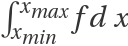, where the integrand f is a function of u, its derivatives and x. ResourceFunction["NVariationalBound"][f,u[x,y,…],{{x,xmin,xmax},…},ut,{a,a0},{b,b0},…] searches for values of the parameters of a trial function of two or more variables. ResourceFunction["NVariationalBound"][{f,g},u[x],{x,xmin,xmax},ut,{a,a0},{b,b0},…] searches for values of the parameters that extremize the ratio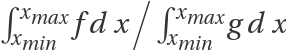, where the integrands f and g are functions of u, its derivatives and x.

## Details and Options

ResourceFunction["NVariationalBound"] returns the extremal value of the functional as well as the optimal parameter values.
ResourceFunction["NVariationalBound"] uses FindMinimum to search for values of the parameters that extremize the functional.
A parameter specification of {a,a0,a1} searches for an extremum using a0 and a1 as the first two values of a, avoiding the use of derivatives.
A parameter specification of {a,a0,amin,amax} searches for an extremum, stopping the search if a ever gets outside the range amin to amax.

## Examples

### Basic Examples

Eigenvalue problem for a third-order ordinary differential equation:

 In:=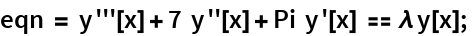In:=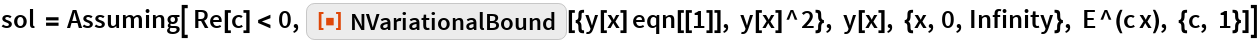Out=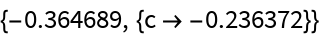The solution fits the equation well in this case:

 In:=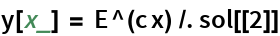Out=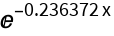In:=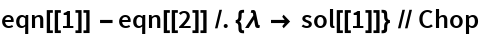Out=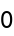In:=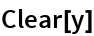## Requirements

Wolfram Language 11.3 (March 2018) or above